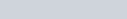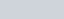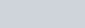A piece of equipment cost a certain factory Rs 60,000.
Question:

A piece of equipment cost a certain factory Rs 60,000. If it depreciates in value, 15% the first, 13.5% the next year, 12% the third year, and so on. What will be its value at the end of 10 years, all percentages applying to the original cost?

Solution:

In the given problem,

Cost of the equipment = Rs 600,000

It depreciates by 15% in the first year. So,

Depreciation in 1 year

$=600000-495000$

$=105000$

$=90000$

It depreciates by 13.5% of the original cost in the 2 year. So,

Depreciation in 2 year $=\frac{13.5}{100}(600000)=81000$

Further, it depreciates by 12% of the original cost in the 3 year. So,

Depreciation in 3 year $=\frac{12}{100}(600000)=72000$

So, the depreciation in value of the equipment forms an A.P. with first term as 90000 and common difference as −9000.

So, the total depreciation in value in 10 years can be calculated by using the formula for the sum of n terms of an A.P,

$S_{n}=\frac{n}{2}[2 a+(n-1) d]$

We get,

$S_{n}=\frac{10}{2}[2(90000)+(10-1)(-9000)]$

$=\frac{10}{2}[180000+(9)(-9000)]$

$=5(180000-81000)$

$=5(99000)$

$=495000$

So, the total depreciation in the value after 10 years is Rs 495000.

Therefore, the value of equipmentSo, the value of the equipment after 10 years is.